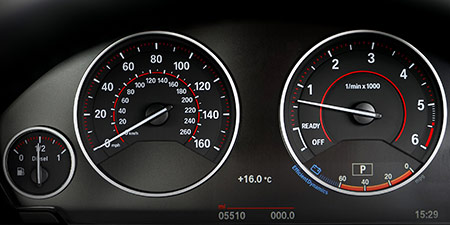# Speed of light to Kilometers per hour (km/h)

Units of speed ﹣ Converter Speed of light to Kilometers per hour

Here you can convert the Speed unit Speed of light into the unit Kilometers per hour and vice versa you can convert Kilometers per hour into Speed of light. By clicking the "Swap units" icon, you will always obtain the desired conversion in the calculation result. With the following calculator you can also calculate any other Speed unit.

## Info about "Speed of light"

Definition: If an object moves at the light speed for one second, it travels a distance of 299,792.458 km or about 300,000 km. This is the light speed that light travels in a vacuum.

This speed is also used to propagate radio signals, which is very important in space travel. For example, a signal from Mars takes about 3 to 20 minutes to reach Earth depending on its actual position. We notice a sunrise on earth about 8 minutes later because the light of the sun arrives to our eyes at that time.

## Info about "Kilometers per hour"

Kilometers per hour is used as a standard unit in transportation, therefore it is used in traffic or to measure the travel speed of vehicles. For example, a commercial aircraft flies at a speed of 1,000 km/h then it covers a distance of 1,000 kilometers per hour.

The unit is defined as follows: An object moving at 1 "km/h" per hour will cover one kilometer. "km/h" is approved to use in the international system of units (SI).

## Formula for the conversion of Speed of light to Kilometers per hour (km/h) and vice versa

The calculation from Speed of light to Kilometers per hour shall be made using the following conversion formula:

Conversion formula Speed of light to Kilometers per hour

Determine the number of Kilometers per hour from Speed of light

Speed of light × 1079252848.8

## Formula for the conversion of Kilometers per hour (km/h) to Speed of light

The calculation from Kilometers per hour to Speed of light shall be made using the following conversion formula:

Conversion formula Kilometers per hour to Speed of light

Determine the number of Speed of light from Kilometers per hour

Kilometers per hour × 9.2656693110598E-10

## Overview table : How many Speed of light are in a Kilometers per hour ?

Speed of light ⇒ Kilometers per hour km/h
0.01   are  10 792 528.48800 km/h
0.02   are  21 585 056.97600 km/h
0.03   are  32 377 585.46400 km/h
0.04   are  43 170 113.95200 km/h
0.05   are  53 962 642.44000 km/h
0.06   are  64 755 170.92800 km/h
0.07   are  75 547 699.41600 km/h
0.08   are  86 340 227.90400 km/h
0.09   are  97 132 756.39200 km/h
0.10   are  107 925 284.88000 km/h
0.20   are  215 850 569.76000 km/h
0.30   are  323 775 854.64000 km/h
0.40   are  431 701 139.52000 km/h
0.50   are  539 626 424.40000 km/h
0.60   are  647 551 709.28000 km/h
0.70   are  755 476 994.16000 km/h
0.80   are  863 402 279.04000 km/h
0.90   are  971 327 563.92000 km/h
1   corresponds to  1 079 252 848.80000 km/h
2   are  2 158 505 697.60000 km/h
3   are  3 237 758 546.40000 km/h
4   are  4 317 011 395.20000 km/h
5   are  5 396 264 244 km/h
6   are  6 475 517 092.80000 km/h
7   are  7 554 769 941.60000 km/h
8   are  8 634 022 790.40000 km/h
9   are  9 713 275 639.20000 km/h
10   are  10 792 528 488 km/h
20   are  21 585 056 976 km/h
30   are  32 377 585 464 km/h
40   are  43 170 113 952 km/h
50   are  53 962 642 440 km/h
60   are  64 755 170 928.00001 km/h
70   are  75 547 699 416.00001 km/h
80   are  86 340 227 904.00001 km/h
90   are  97 132 756 392.00001 km/h
100   are  107 925 284 880.00001 km/h
200   are  215 850 569 760.00003 km/h
300   are  323 775 854 640.00006 km/h
400   are  431 701 139 520.00006 km/h
500   are  539 626 424 400.00012 km/h
600   are  647 551 709 280.00012 km/h
700   are  755 476 994 160.00012 km/h
800   are  863 402 279 040.00012 km/h
900   are  971 327 563 920.00012 km/h
1 000   are  1 079 252 848 800.00024 km/h

## Overview table : How many Kilometers per hour are in a Speed of light ?

Kilometers per hour km/h ⇒ Speed of light
0.20 km/h  are  0.0000000001
0.30 km/h  are  0.0000000002
0.40 km/h  are  0.0000000003
0.50 km/h  are  0.0000000004
0.60 km/h  are  0.0000000005
0.70 km/h  are  0.0000000006
0.80 km/h  are  0.0000000007
0.90 km/h  are  0.0000000008
1 km/h  corresponds to  0.0000000009
2 km/h  are  0.0000000018
3 km/h  are  0.0000000027
4 km/h  are  0.0000000037
5 km/h  are  0.0000000046
6 km/h  are  0.0000000055
7 km/h  are  0.0000000064
8 km/h  are  0.0000000074
9 km/h  are  0.0000000083
10 km/h  are  0.0000000092
20 km/h  are  0.0000000185
30 km/h  are  0.0000000278
40 km/h  are  0.0000000370
50 km/h  are  0.0000000463
60 km/h  are  0.0000000555
70 km/h  are  0.0000000648
80 km/h  are  0.0000000741
90 km/h  are  0.0000000833
100 km/h  are  0.0000000926
200 km/h  are  0.0000001853
300 km/h  are  0.0000002779
400 km/h  are  0.0000003706
500 km/h  are  0.0000004632
600 km/h  are  0.0000005559
700 km/h  are  0.0000006486
800 km/h  are  0.0000007412
900 km/h  are  0.0000008339
1 000 km/h  are  0.0000009265

## Source information

As source for the information in the 'Units of speed' category, we have used in particular:

## Last update

This page of the 'Units of speed' category was last edited or reviewed by Stefan Banse on February 19, 2023. It corresponds to the current status.

### Changes in this category "Units of speed"

• November 9, 2020: Publication of the Speed converter